# Test: Square Root And Cube Root- 2

## 10 Questions MCQ Test Quantitative Aptitude for Banking Preparation | Test: Square Root And Cube Root- 2

Description
Attempt Test: Square Root And Cube Root- 2 | 10 questions in 10 minutes | Mock test for UPSC preparation | Free important questions MCQ to study Quantitative Aptitude for Banking Preparation for UPSC Exam | Download free PDF with solutions
QUESTION: 1

### How many two-digit numbers satisfy this property : The last digit (units digit) of the square of the two-digit number is 8 ?

Solution:

A number ending in 8 can never be a perfect square.
12 = 1
22 = 4
32 = 9
42 = 16
52 = 25
62 = 36
72 = 49
82 = 64
92 = 81

QUESTION: 2

### if a = 0.2917, then the value of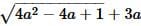is :

Solution: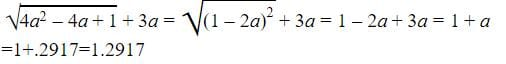QUESTION: 3

### A group of students decided to collect as many paise from each member of group as is the number of members. If the total collection amounts to Rs. 59.29, the number of the member is the group is:

Solution:

Money collected = (59.29 x 100) paise = 5929 paise.
∴ Number of members = √5929 = 77.

QUESTION: 4

if √7 = 2.645, then find the value of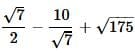Solution: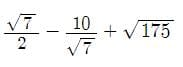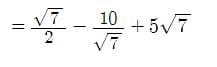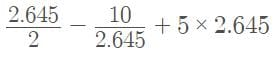= 1.3225 - 3.78071 + 13.225
= 10.76679 ≈ 10.77

QUESTION: 5

What is smallest number with which 5400 may be multiplied so that the product is perfect cube?

Solution:

Find prime factors of 5400

5400=2×3×3×3×2×2×5×5

If we group them in the group of 3

5400=(2×2×2)×(3×3×3)×5×5

Here to make group of 3 for 5

We have to multiply 5400 by 5.

QUESTION: 6

The square root of (14 + 2√13)(14 - 2√13) is

Solution:

As we know that  (a²-b²) = (a+b) (a-b)
Therefore 14² - 2²x13 = 144.
So √144 = 12.

QUESTION: 7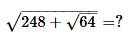Solution:

= [√248+√64]
= √248+8
= √256
= 16

QUESTION: 8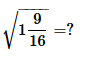Solution:

√1 9/16
= √25/16
= 5/4
= 1 1/4.

QUESTION: 9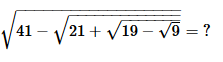Solution:

√41-√21+√19-√9
= √41-√21+√19-3
=√41-√21+√16
=√41-√21+4
=√41-√25
=√41-5
=√36
=6

QUESTION: 10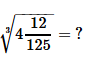Solution:

Cube root of (512)/125
= 8/5
Convert it into mixed fraction, we get
= 1 3/5Use Code STAYHOME200 and get INR 200 additional OFF Use Coupon Code